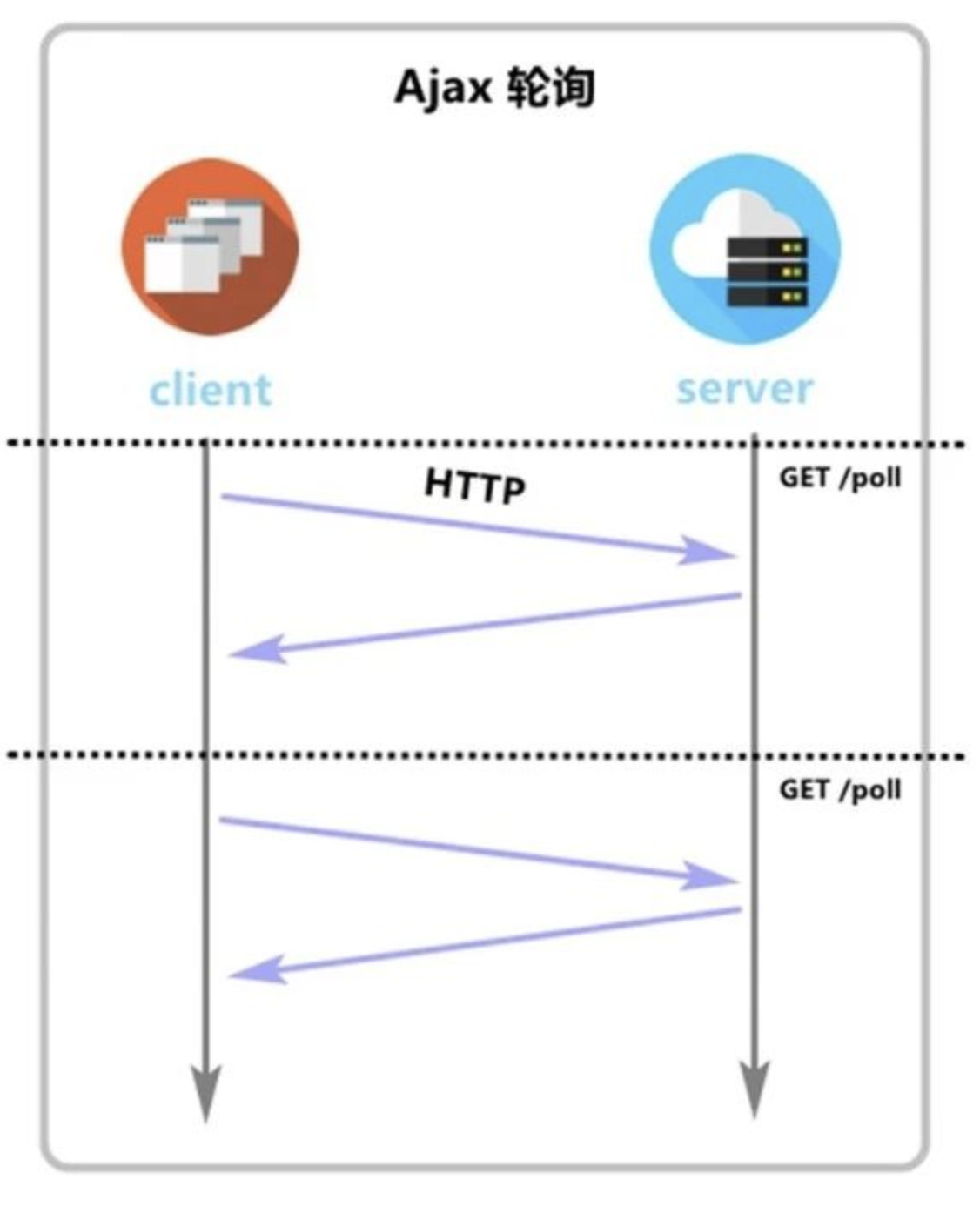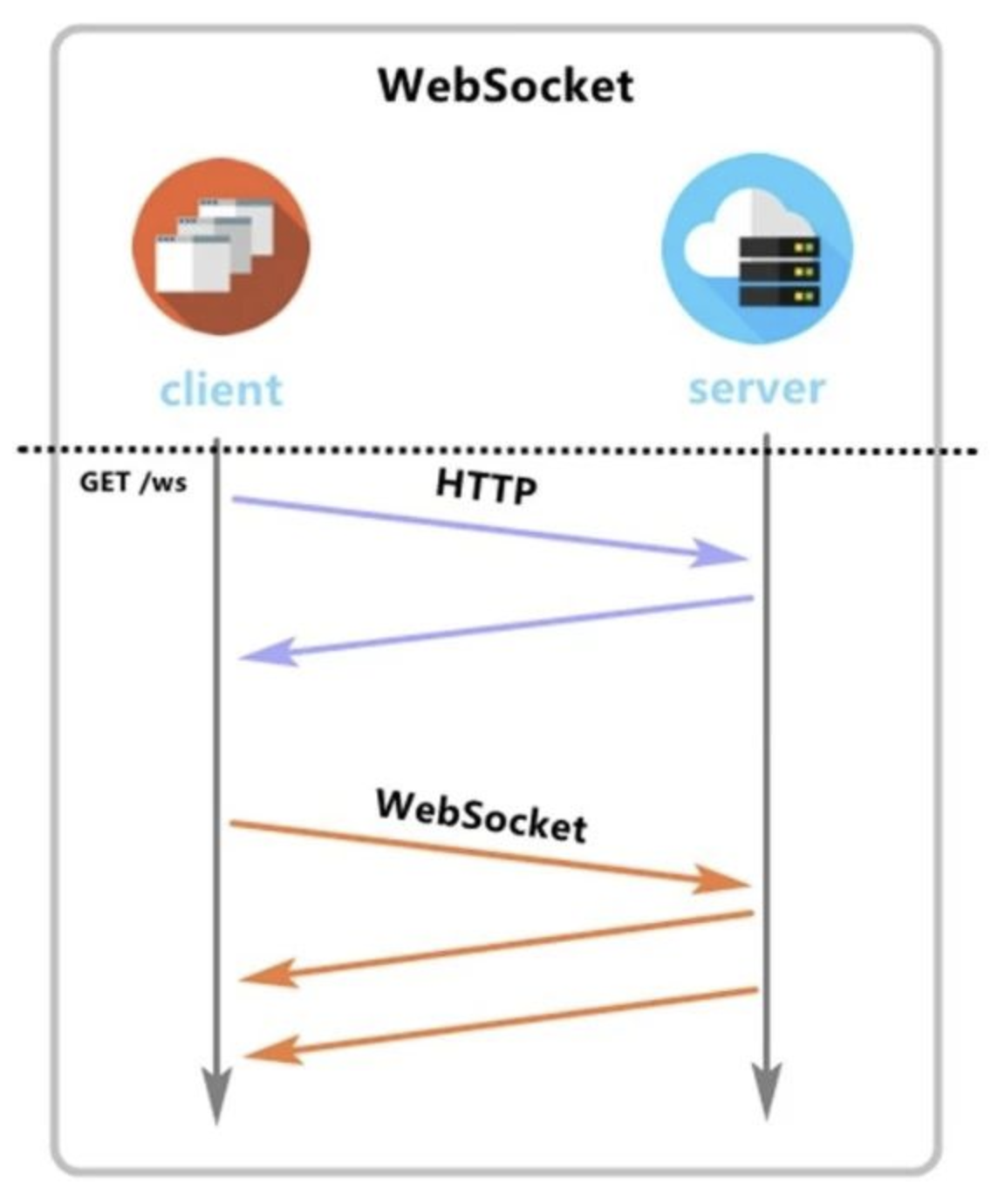# Ajax轮询和SSE服务器推送数据与websocket模式的区别性学习

## Ajax轮询

Node后端

``````const Koa = require("koa");
const static = require("koa-static");
const Router = require("koa-router");
const mysql2 = require("mysql2");
const koaBody = require("koa-body");
let app = new Koa();
app.use(static(__dirname+"/static"));
app.use(koaBody());
//连接数据库
const connection = mysql2.createConnection({
host:"localhost",
user:"root",
database:"js04"
})
let router = new Router();
//访问此路由进行获取数据
router.get("/getData",async ctx=>{
let [rows] = await connection.promise().query("SELECT * FROM message");
ctx.body = rows;
})

app.use(router.routes());
app.listen(3000);``````

``````\$.ajax({
//访问路由
url: "/getData",
success(res) {
//res 就是回的数据
console.log(res);
}
})``````

``````    setInterval(() => {
render();
}, 500);
``````## SSE (server send event) 服务器推送数据

• 设置头部

`"Content-type","text/event-stream"`

• 返还数据格式

`data:` 声明数据开始

`\r\n\r\n ` 标志数据结尾

``````const http = require("http");
const fs = require("fs");
// res.write();  res.end();
let server = http.createServer((req,res)=>{
let url = req.url;
//路由返回页面
if(url=="/"){
res.end(data);
}else if(url=="/sse"){
// 服务端端定时推送数据到客户端；
setInterval(() => {
res.write("data:时间是"+new Date()+"\r\n\r\n");
}, 1000);
}
})
server.listen(4000);
``````

1. open：当成功建立连接时产生
2. message：当接收到消息时产生
3. error：当出现错误时产生
``````let source = new EventSource("/sse");
source.onopen = function(){
console.log("连接成功....");
//- 0 CONNECTING (0)
//- 1 OPEN (1)
//- 2 CLOSED (2)
}
source.onmessage = function(e){
console.log("获取到的数据是：",e.data);
document.querySelector(".exchange").innerHTML = e.data;
}
source.onerror = function(err){
return console.log(err);
}``````

• CONNECTING (0)
• OPEN (1)
• CLOSED (2)

## websocket

WebSocket 是 HTML5 开始提供的一种在单个 TCP 连接上进行全双工通讯的协议

ws是一种易于使用，快速且经过全面测试的WebSocket客户端和服务器实现的方法。

### 安装

``npm install ws``

### 简单使用

``````var WebSocketServer = require('ws').Server,
wss = new WebSocketServer({ port: 8181 });
wss.on('connection', function (ws) {
console.log('client connected');
ws.on('message', function (message) {
//监听接收的数据
console.log(message);
});
// setInterval(() => {
let somedata = {
name:"张三",
age:20
}
//发送数据
ws.send(JSON.stringify(somedata));
// }, 1000);
});``````

``var ws = new WebSocket("ws://localhost:8181");``

``ws.onopen = function () {}``

``ws.send("客户端数据");``

``ws.close();``

``````ws.onmessage = function(e){
// console.log(e.data);
}``````

``````// 握手协议；
var ws = new WebSocket("ws://localhost:8181");
ws.onopen = function(){
console.log("连接成功....");
}
ws.onmessage = function(e){
// console.log(e.data);
console.log(JSON.parse(e.data));
}
function sub(){
ws.send("发送到服务端的数据");
}``````